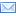#Difference Between Similar Terms and Objects

# Difference Between Algebra and CalculusAlgebra vs Calculus

Mathematics is an integral part of our everyday lives. Every day we are faced with numbers, from the moment we wake up and think about how many people to cook for, how much money to spend on necessary items and how long it would take to reach the office.

This is why we are taught mathematics in school, to make life easier for us and to help us understand numbers.  There are many branches of mathematics, two of which are Algebra and Calculus.

Both are branches of mathematics that are very useful in people’s lives.  They are used for different calculations and like all the other branches of mathematics such as geometry and trigonometry, they are interrelated and are sometimes used individually or together to solve mathematical problems.

Algebra

Algebra is one of the main branches of pure mathematics that studies the rules of operations and relations and their application to solve equations. It is one of the oldest branches of mathematics.

It studies the terms, constructions and concepts arising from mathematical operations and it also deals with the study of polynomials, equations, and algebraic structures.

Algebra studies what happens when different operations are devised and used for things other than numbers.  It uses letters to represent numbers which are combined according to the rules of mathematics.

There are several categories of algebra, namely:

1. Elementary algebra, which introduces the concept of variables representing numbers in secondary education.
2. Abstract algebra or modern algebra, wherein algebraic structures like groups, rings, and fields, are defined and investigated.
3. Universal algebra, the study of common properties in algebraic structures.
4. Linear algebra, the study of the properties of vector spaces and matrices.
5. Algebraic number theory, the study of the properties of numbers.
6. Algebraic systems, the study of the theory of abstraction in algebra.
7. Algebraic geometry, the use of abstract algebra in geometry.
8. Algebraic combinatorics, the use of abstract algebraic methods to study combinatorial questions.

Calculus

Calculus is a branch of mathematics that studies change. Â It focuses on limits, functions, derivatives, integrals and infinite series. Â It is the foundation to more advanced courses in mathematics and is widely used in science, economics, engineering, physical and computer science, business, medicine and other fields wherein an optimal solution is needed.

It is used to solve mathematical problems that cannot be solved by algebra and helps in determining the rate a variable will change in relation to others. Â It is used in determining the cost of production, supply and demand, temperature, pressure and other mathematical problems that need to be analyzed.

Calculus has two major branches, differential and integral.

Summary:

1. Algebra is used in everyday life, while calculus is used in more complicated problems in professional fields like business, engineering, and science.
2. Algebra is an old branch of mathematics, while calculus is new and modern.
3. Algebra is easier to understand, while calculus is very complex.
4. Algebra is the study of relations, while calculus is the study of change.

### Search DifferenceBetween.net :

Custom Search

Help us improve. Rate this post!(4 votes, average: 5.00 out of 5)Loading...Email This Post : If you like this article or our site. Please spread the word. Share it with your friends/family.

1. Algebra is the oldest branch of the Mathematics.It helps Mathematics that studies operaratations and relations to solve equations and thesis.

2. Caculus is the branch of Mathematics that studies changes.it helps to solve the applications of Derivatives,Limits, Functions, Integrals and infinite series.It is to more advance foundation course in Mathematics and is widely used in sciences, Economics, engineering, physical and computer-science,business and medicines.

3. Nice

Please note: comment moderation is enabled and may delay your comment. There is no need to resubmit your comment.

Articles on DifferenceBetween.net are general information, and are not intended to substitute for professional advice. The information is "AS IS", "WITH ALL FAULTS". User assumes all risk of use, damage, or injury. You agree that we have no liability for any damages.

See more about : , ,